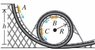# Tangential Acceleration of park ride

• Peach
Thanks for the help!In summary, the car in an amusement park ride rolls without friction around the track shown in the figure. At point C, the car is at the end of a horizontal diameter. The car starts from rest at point A at a height h above the bottom of the loop. The tangential acceleration of the passengers is r*alpha. If the car starts at height h = 72.0 m and the radius is R = 16.0 m, compute the tangential acceleration of the passengers when the car is at point C.f

## Homework Statement

A car in an amusement park ride rolls without friction around the track shown in the figure View Figure . It starts from rest at point A at a height h above the bottom of the loop. Treat the car as a particle.

If the car starts at height h = 72.0 m and the radius is R = 16.0 m, compute the tangential acceleration of the passengers when the car is at point C, which is at the end of a horizontal diameter.

Don't know...

## The Attempt at a Solution

This is part of a problem and I've already solved the first three. I have the radial acceleration, the velocity at B, and the velocity at C. But I don't know how to find the tangential acceleration at C. I've tried Newton's laws already to find the acceleration so that I can use a^2 = a_rad^2 + a_tan^2 but I don't have time or distance between C and B. Can someone pls give me a nudge in the right direction? I've been struggling over this problem for two hours already. ><

#### Attachments

•yf_Figure_7_32.jpg
11.1 KB · Views: 625
Hey, how did you get Part A? I have managed to get the other parts except for the Tangential Acc part but I'm working on it and making sure my calculations are right. When i get it i'll tell you how I got it but can you help me with the first part?

How did you get the other parts without doing part A?Use the conserved energy law. K_1 + U_1 = K_2 + U_2 right? Point 1 should be at the top of the roller coaster with height h. Point 2 should be at B. Then just plug and chug. ^^

it's late lol, soo my mind is working in funky mode. while waiting I did part a lol. Going to do part D now, but might get back to it tomorrow. tired x_x

I understand. ^^; I'm not getting any of this stuff either. Been stuck on a problem for hours already. Going to post it now.

BUMP. Pls help...still stuck, it's not getting through. :x

Ok, what is the exact definition of Tangential Acceleration?

I spent about an hour researching to see if I could find an formula and I found one but when I found the answer I realized it was right under my nose the whole time.

*hint: You didn't post all the information in the problem. The missing information is the key to finding the answer.

I didn't post part A right? But what does the minimum height have to do with this...? I found a formula for tangential acceleration too, not sure if it's the same as yours too. It's r*alpha but I can't apply that to this. At least I don't think so. o_o

Uh tangential acceleration, it's in the direction of the velocity vector, that's how the speed increase.

If the car starts at height h = 60.0 m and the radius is R = 15.0 m, compute the tangential acceleration of the passengers when the car is at point C, which is at the end of a horizontal diameter.
Take the free fall acceleration to be g = 9.80 m/s^2.

That is my question.

The Tangential Acceleration is down, the normal force at point C is horizontal, there is no friction so the only downward force is gravity.

Like i said, the missing piece of information is the key to the answer. I spent sooo much time looking at the complexity that I forgot the base of physics will always be the simplicity.

Omg, the missing piece you were talking about was the gravity acceleration. I thought it was the height. Eh. This officially makes me feel very very dumb. o_o But yeah, thanks for helping me. I spent so much time on this problem too. ><

No need to feel dumb, it took me HELLA long to figure that out lol. It wasn't until my boyfriend pointed that out that I realized what it was ha ha. I always forget not to overlook the obvious things in focus on the complexity